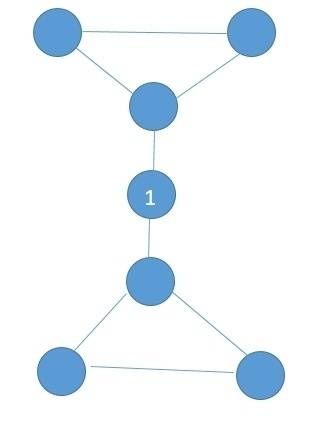# Graph theory proof

## Homework Statement

Exercise 0.1. Suppose that G is a finite graph all of whose vertices has degree two or greater. Prove that a cycle passes through each vertex. Conclude that G cannot be a tree.

## The Attempt at a Solution

If every vertex in a graph G has degree two or greater, then there is always a way to get in and out without using the same edge. If we start at any vertex and try to traverse the graph by using the rule of getting in and out and not using the same edge, eventually we will realize that we can't stop, so this implies that there is a cycle. Therefore G can't be a tree.

I am new to this types of proofs, so I need feedback.

Last edited:

Samy_A
Homework Helper

## Homework Statement

Exercise 0.1. Suppose that G is a finite graph all of whose vertices has degree two or greater. Prove that a cycle passes through each vertex. Conclude that G cannot be a tree.

## The Attempt at a Solution

If every vertex in a graph G has degree two or greater, then there is always a way to get in and out without using the same edge. If we start at any vertex and try to traverse the graph by using the rule of getting in and out and not using the same edge, eventually we will realize that we can't stop, so this implies that there is a cycle. Therefore G can't be a tree.

I am new to this types of proofs, so I need feedback.
Consider the following graph:Apply your proof to vertex 1. Does it show that there is a cycle through vertex 1?

haruspex
Homework Helper
Gold Member
2020 Award
Consider the following graph:
View attachment 94303
Apply your proof to vertex 1. Does it show that there is a cycle through vertex 1?
That's not just a counterexample to the proof, it's a counterexample to the given claim.

Samy_A
Homework Helper
That's not just a counterexample to the proof, it's a counterexample to the given claim.
I know, it was a not too subtle hint.The "each vertex" in the statement of the exercise must be an error, and the exercise was probably something like: "prove that the graph contains a cycle".

haruspex
I know, it was a not too subtle hint.